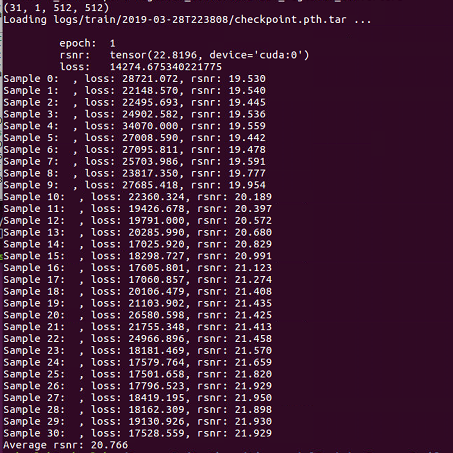# Weird performance when load pre-trained model

Hi,
I got a weird performance when I loaded a pre-trained model.The model was trained one epoch, the learned parameters are saved, as well as the measure value of average ‘rsnr’ and ‘loss’ on validation dataset. As you can see, when I load the saved model to test it on the validation dataset, the average rsnr is quit different, not the same as in the saved model.

I have checked a lot but I failed to fix this. I’m sure the loaded model was in `eval` mode, the measures were the same function and the input of the network was the same.

Thanks a lot for your suggestions.

Is the ‘rsnr’ value varying everytime you load the same model or it stays same?

It stays same every time I load it.

I see that even the average of the test-loss is well-off from what you have printed from the model. Are you sure that the ‘rsnr’ value from the model is from “test” and not “train”?
Further, we would need to look at the code to spot the issue I guess.

Sorry about that I can not show all the codes. Here are some snippets.

``````# This is the snippet in main function of training network
best_measure = 0.
t = 0.
for e in range(1, numEpochs+1):
# Update the learning rate
scheduler.step()
# Here `train` and `test` is function for training and testing network, respectively.
t = train(e, t)
loss, c_measure, data, logits, label = test(e, t)
torch.save({'epoch': e,
'state_dict': model.state_dict(),
'rsnr': c_measure,
'loss': loss,
'optimizer': optimizer.state_dict()}, save_model_path)
if c_measure >= best_measure:
shutil.copyfile(save_model_path, best_model_path)
best_measure = c_measure
``````
``````# This is the test function code
def test(epoch, ttot):
model.eval()
test_loss = AverageMeter()
test_measure = AverageMeter()
for batch_idx, (data, target) in enumerate(val_loader, 1):
model.eval()
# where are we.
dataset_size = len(train_set)
iteration = (epoch-1) * (dataset_size // config['batch-size']) + batch_idx + 1

data, target = data.to(device), target.to(device)
logits = model(data)

loss = criterion(logits, target)
l_measure = rsnr(logits, target)
test_measure.update(l_measure, 1)
test_loss.update(loss.data.item(), data.size(0))

testing_logger(epoch, test_loss.avg, test_measure.avg, optimizer)
print('[Epoch %2d] Average test loss: %.3f, Average test RSNR: %.3f'
%(epoch, test_loss.avg, test_measure.avg))

return test_loss.avg, test_measure.avg, data, logits, target
``````

And the following is the snippets for testing the network after loading the saved model

``````ave_measure = 0
model.eval()
for i in range(nums):
model.eval()
data, target = torch.from_numpy(sparse[i,0:]).float().unsqueeze(0).to(device), \
torch.from_numpy(label[i,0:]).float().unsqueeze(0).to(device)
logits = model(data)
loss = criterion(logits, target) # `criterion` is the loss function
l_measure = rsnr(logits, target) # `rsnr` is the measure function
print('Sample %d:  , loss: %.3f, rsnr: %.3f'%(i, loss.item(), l_measure))
ave_measure = ave_measure + l_measure
print('Average rsnr: %.3f'%(ave_measure/nums))

``````

Thank you, Arul.

Edit: I posted the `test` funtion.

I’m sure the ‘rsnr’ value is from test dataset.

Hi, I have fixed the problem. Finally it turns out there is something wrong with the data.
Thank you.